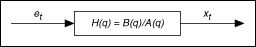# Building Autoregressive-Moving Average Models (Advanced Signal Processing Toolkit)

LabVIEW 2014 Advanced Signal Processing Toolkit Help

Edition Date: June 2014

Part Number: 372656C-01

»View Product InfoDownload Help (Windows Only)

Autoregressive-moving average (ARMA) models are more accurate than autoregressive (AR) models in approximating the response of linear systems with zeros in the response transfer function. The following equation defines an ARMA model with an AR order of n and the moving average (MA) order of m:

xt + a1xt–1 + a2xt–2 + ... + anxt–n = et + b1et–1 + ... + bmet–m

where [1, a1, a2, ..., an] are the AR coefficients, and [1, b1, a2, ..., bm] are the MA coefficients.

You can rewrite the previous equation more concisely as follows:

A(q)xt = B(q)et

where A(q) is the AR operator and B(q) is the MA operator defined as follows:

B(q) = 1 + b1q–1 + b2q–2 + ... + bmq–m

The following figure shows a diagram that uses an ARMA model to describe a linear system. You can consider a time series xt as being the response of a system whose transfer function H(q) is B(q)/A(q). The stimulus of the system is et.H(q) contains both zeros and poles. The roots of the polynomial A(q) are the poles of H(q). The roots of the polynomial B(q) are the zeros of H(q). The ARMA model uses poles and zeros to describe a system. Therefore, comparing with the AR model, the ARMA model can give a more accurate description of the dynamic characteristics of a physical system that generates time series, if the system has an ARMA response. However, because the MA term introduces nonlinearities in the model estimation, you cannot use an analytic form to solve the model coefficients. An iterative nonlinear optimization procedure is required, which could find a sub-optimal solution erroneously instead of finding the globally optimal solution.

You also can estimate a vector ARMA (VARMA) model for a multivariate time series. The resulting VARMA model of a multivariate time series is the coefficients matrix. However, you cannot obtain the dynamic characteristics of a multi-output system directly from the coefficients matrix of the VARMA model. You must convert the model coefficients matrix into a state transition matrix in a stochastic state-space model. By computing the eigenvalues of a state transition matrix, you can obtain the poles of the system that generates the corresponding multivariate time series. You then can obtain the dynamic characteristics of the system from the poles.

An MA model is a special case of the ARMA model that does not contain poles. The MA model describes a time series according to the following equation:

xt = B(q)et

Use the TSA MA Modeling VI to build MA and VMA models for univariate or multivariate time series, respectively. Use the TSA ARMA Modeling VI to build ARMA and VARMA models. You also can use the TSA ARMA Modeling VI to build MA models by setting the AR order to zero.Note  An AR model also is a special case of the ARMA model with the MA order equals zero. However, the TSA ARMA Modeling VI is not suitable for estimating AR models. Use the TSA AR Modeling VI to estimate an AR model, because the TSA AR Modeling VI is more efficient than the TSA ARMA Modeling VI.

You also can use the Time Series Modeling Express VI to build MA, ARMA, and VARMA models, interactively.

## Selecting an Appropriate ARMA Order

Before estimating the ARMA model coefficients of a time series, you need to specify a suitable order for the model. Generally, you specify an AR order of n and an MA order of n – 1, where n is an integer. If you know the order of the response of the system that generates the time series, you can use that order directly. If you do not know the order, you can try orders from low to high to identify the lowest order in which the prediction error is sufficiently white. You can use the TSA Whiteness Test VI to perform whiteness tests on the residual prediction error, by computing auto-correlation and the corresponding confidence limit for the auto-correlation.

The variance of the prediction error also indicates if the ARMA model at a specified order fits the time series well. The smaller the variance of the prediction error is, the better the estimated model fits the time series. However, the variance of the prediction error for ARMA models decreases monotonically with order. Unnecessarily high orders introduce spurious artifacts in the resulting response if the measured time series is contaminated with noise or distortion.

The changes in the variance of the prediction error that result from changes in the model order can indicate dynamic characteristics of a physical system that generates time series. This property is helpful in vibration monitoring applications.

Refer to the Engine Knocking Monitor VI in the labview\examples\Time Series Analysis\TSAApplications directory for an example that demonstrates the application of autoregressive-moving average (ARMA) modeling in examining the condition of an engine.